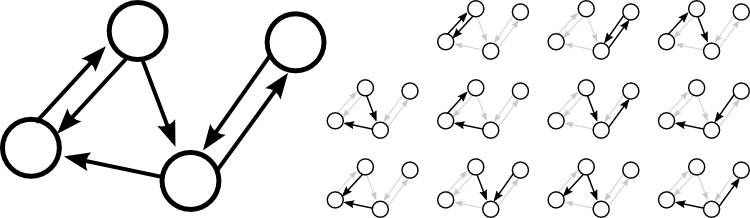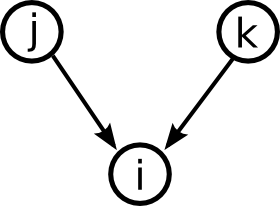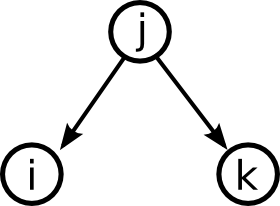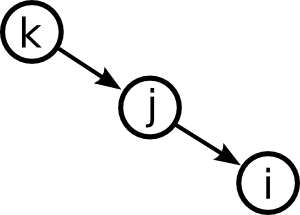# Math Insight

### Generating networks with a desired second order motif frequency

One way to add structure beyond the Erdös-Rényi random network (ER random network) is to generate ensembles of networks with a prescribed degree distribution. Here we describe another approach: modulating the frequencies of small network motifs in directed graphs.

#### Network motifs

Network motifs are patterns of connections within a network. For example, the simplest motif consists of a single edge from one node to another.Moving up one step in complexity, we can look at motifs involving two edges. If we restrict ourselves to connected motifs (excluding the motif with two edges connecting distinct pairs of nodes), there are four motifs with two edges, which we can label as the reciprocal, convergent, divergent, and chain motifs.

One can characterize a network by counting the relative frequency of these five motifs. Here, each motif represents a pattern of the one or two edges shown without regard to the presence or absence of any of the edges not shown.

For example, the following four node network has six edges, so the single-edge motif occurs six times. The total possible number of connections is $4\cdot 3 = 12$, so the network contains 50% of the possible edges.As highlighted on the right, the network contains two reciprocal motifs, five chain motifs, two convergent motifs, and two divergent motifs. The total number of possible reciprocal motifs is $4 \cdot 3/2 = 6$, so the network contains 1/3 of the possible reciprocal motifs. The total number of possible chain motifs is $4 \cdot 3 \cdot 2 = 24$, so the network contains $5/24$ of the possible chain motifs. For both convergent and divergent motifs, the total possible number is $4 \cdot 3 \cdot 2/2 = 12$; the network contains 1/6 of the possible convergent and 1/6 of the possible divergent motifs.

#### Generating networks with given expected motif frequencies

One can view the ER random network, as a probability distribution parametrized by the expected relative frequency of the single-edge motif, which is the same as the connection probability.We could specify that, for any pair of nodes $i$ and $j$, the probability of the motif containing a connection from $j$ to $i$ is \begin{gather} \Pr(A_{ij}=1)=E(A_{ij})=p, \label{probedge} \end{gather} where the expected value $E(\cdot)$ is computed over the probability distribution of the adjacency matrix, equation (1) of the random network page.

In the ER random network, the second order connectivity motifs will occur, too. But, we cannot independently specify their frequencies. Since all edges occur independently with probability $p$, any pair of edges (such as the two-edge motifs) must occur with probability $p^2$. (The probability of two independent random variables is the product of their individual probabilities.)

Given that real world networks motif frequencies differ from the ER prediction, we'd like to generate networks where the two-connection motifs occur at frequencies other than $p^2$. We introduce four more parameters, $\alpha_{\text{recip}}$, $\alpha_{\text{conv}}$, $\alpha_{\text{div}}$, and $\alpha_{\text{chain}}$, through which to specify the frequencies of the reciprocal, convergent, divergent, and chain motifs, respectively. We define each $\alpha$ to indicate deviation from the ER model, so if an $\alpha$ is zero, the corresponding motif will appear with probability $p^2$. The definitions of the four $\alpha$ parameters in terms of the probability of the respective motif are as follows.$$\Pr(A_{ij}\nobreak{=}1\nobreak{,}A_{ji}\nobreak{=}1) = p^2(1+\alpha_{\text{recip}})$$$$\Pr(A_{ij}\nobreak{=}1\nobreak{,}A_{ik}\nobreak{=}1)=p^2(1+\alpha_{\text{conv}})$$$$\Pr(A_{ij}\nobreak{=}1\nobreak{,}A_{kj}\nobreak{=}1)= p^2(1+\alpha_{\text{div}})$$$$\Pr(A_{ij}\nobreak{=}1\nobreak{,}A_{jk}\nobreak{=}1)=p^2(1+\alpha_{\text{chain}})$$

As we manipulate the expected frequencies of the second order connection motifs, we are still holding the expected frequency of the single-edge motif at $p$. We cannot increase the frequency of any two-edge motif by adding additional edges, or that would increase the frequency of the single-edge motif. Instead, if we would like to increase the frequency of the reciprocal motif, for example, we need to increase the probability that both $A_{ij}$ and $A_{ji}$ are one and simultaneously increase the probability that both $A_{ij}$ and $A_{ji}$ are zero (while decreasing the one edge occurs without the other). We must do this in a way to keep the probability of all single-edge motifs at $p$.

We can express the fact that the $\alpha$'s simply must increase the coordination between their edges by writing them in terms of the covariances between edges. Using equation \eqref{probedge} for single-edge probability, one can rewrite the definitions for the $\alpha$'s as normalized covariances. \begin{align*} \alpha_{\text{recip}} &= \frac{\text{cov}(A_{ij},A_{ji})}{E(A_{ij})E(A_{ji})} &\alpha_{\text{conv}}&=\frac{\text{cov}(A_{ij},A_{ik})}{E(A_{ij})E(A_{ik})}\\ \alpha_{\text{div}}&=\frac{\text{cov}(A_{ij},A_{kj})}{E(A_{ij})E(A_{kj})} &\alpha_{\text{chain}}&=\frac{\text{cov}(A_{ij},A_{jk})}{E(A_{ij})E(A_{jk})} \end{align*}

For ER random networks, it is a simple matter to generate a network with given edge probability, as we can generate the random number for each edge independently using equation \eqref{probedge}. However, when we break this independence by adding correlations among the edges through nonzero $\alpha$'s, the process of generating a network with the required statistics is more subtle. It turns out one can define a random network model based on these statistics, the SONET (second order network) model1 and sample networks with different values of the $\alpha$'s. In this way, we obtain a probability distribution for the network parametrized by the first order statistic $p$ and the second order statistics $\alpha_{\text{recip}}$, $\alpha_{\text{conv}}$, $\alpha_{\text{div}}$, and $\alpha_{\text{chain}}$.